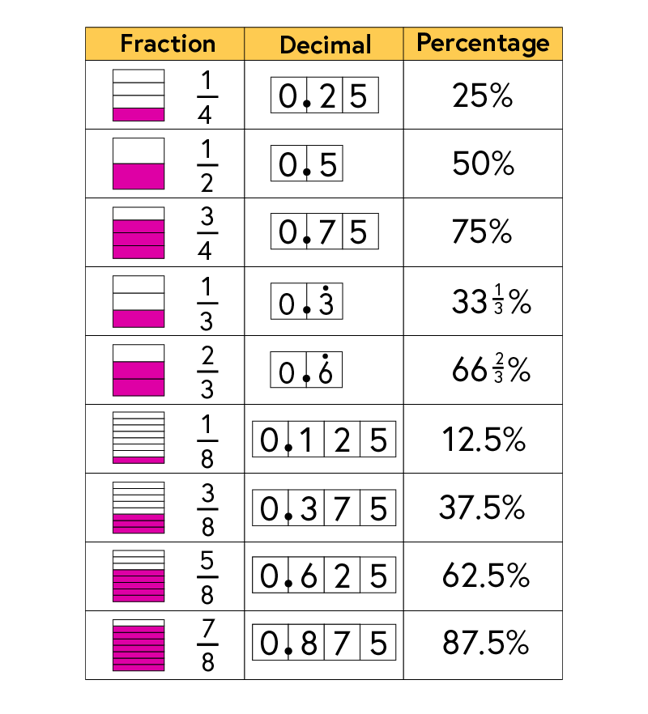## Want to keep learning?

This content is taken from the National STEM Learning Centre's online course, Maths Subject Knowledge: Fractions, Decimals, and Percentages. Join the course to learn more.
3.9

# Revisiting percentages

In week two we saw that a number can be expressed as a fraction, as a decimal and as a percentage.

Here is a recap of some of the most common equivalences.We are familiar with the fact that a half of 30 is 15. It is important that students appreciate that this means that 30 multiplied by 0.5 is equal to 15 and that 50% of 30 is equal to 15. In each case we are saying the same thing in a different way.

Students will be expected to be able to calculate a percentage of an amount.

In the next step we explore methods to find a percentage of an amount which develop mathematical understanding in addition to procedural fluency.